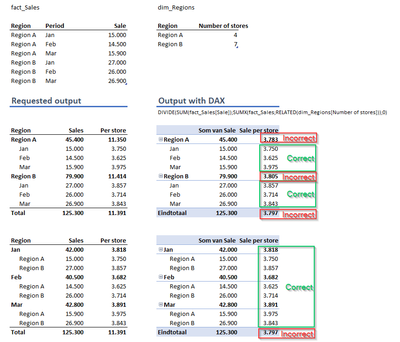cancel
Showing results for
Did you mean:Regular Visitor

## DAX formula weighted average support requested

Hello community,

Can someone help me with a DAX formula to calculate average sales per store per period and per region as in the below example?

Even after reading many questions on the same topic, I can't get it to work. My DAX attempt in the screenshot comes close but the aggregated results on period level and store level are ok, but not on total and not on Region level.

Here is the model and my DAX attempt (DIVIDE(SUM(fact_Sales[Sale]);SUMX(fact_Sales;RELATED(dim_Regions[Number of stores]));0))Thanks in advance for any support.

Michel

1 ACCEPTED SOLUTIONSuper User

Assuming you're using dim_Regions[Region] in your visual, you should be able to define the ratio as:

``````Sales per store =
DIVIDE (
SUM ( fact_Sales[Sale] );
SUM ( dim_Regions[Number of stores] );
0
)``````
3 REPLIES 3Regular Visitor

Hello AlexisOlson,

Thanks for your reply, this is indeed the (a?) solution that solves the problem from my question. It shows me again that I'm not used to thinking in a DAX way, in Excel I would have solved the issue probably much faster.Super User

DAX definitely takes some getting used to. I like it much better than Excel formulas now though (which tend to be difficult to read).Super User

Assuming you're using dim_Regions[Region] in your visual, you should be able to define the ratio as:

``````Sales per store =
DIVIDE (
SUM ( fact_Sales[Sale] );
SUM ( dim_Regions[Number of stores] );
0
)``````